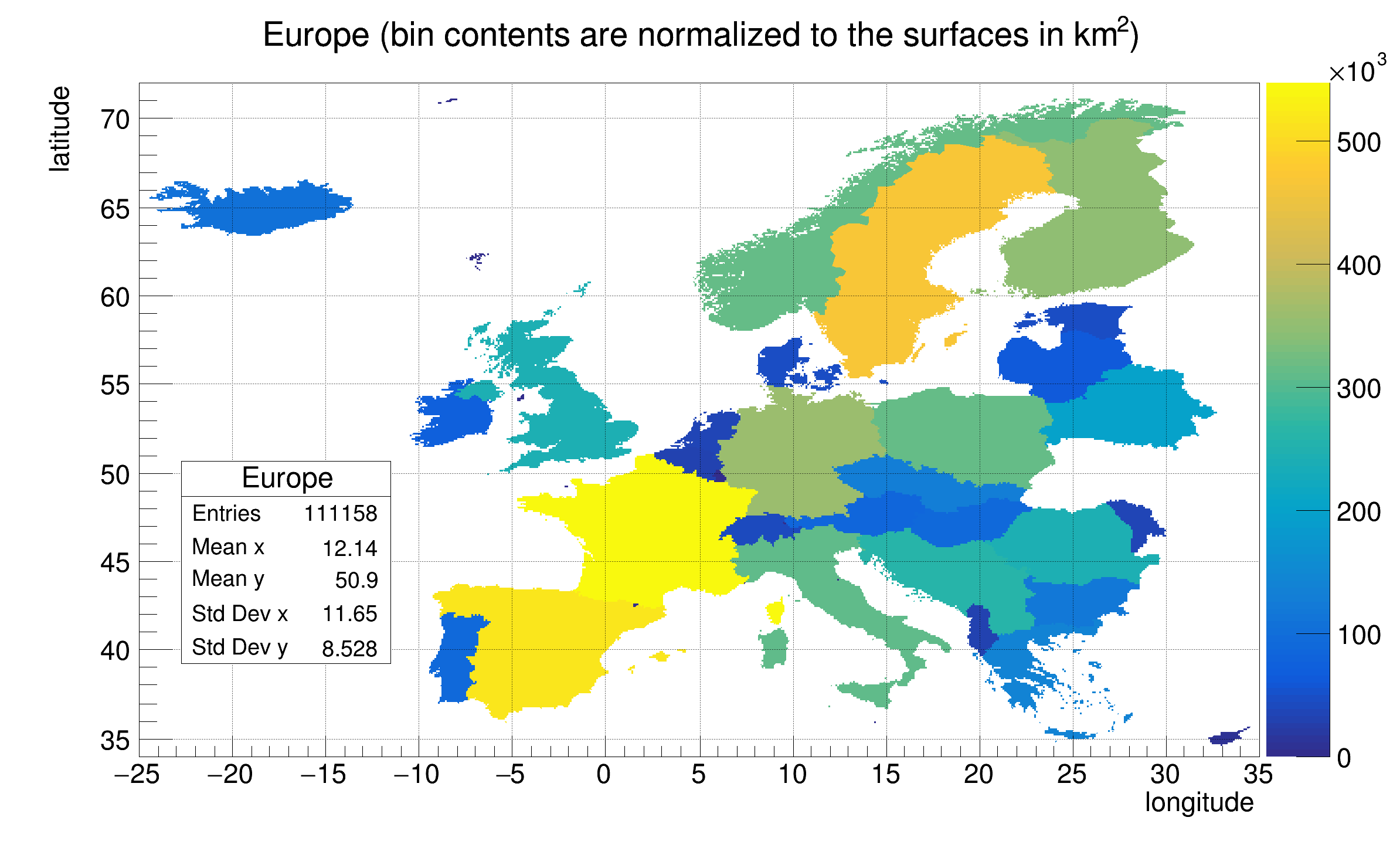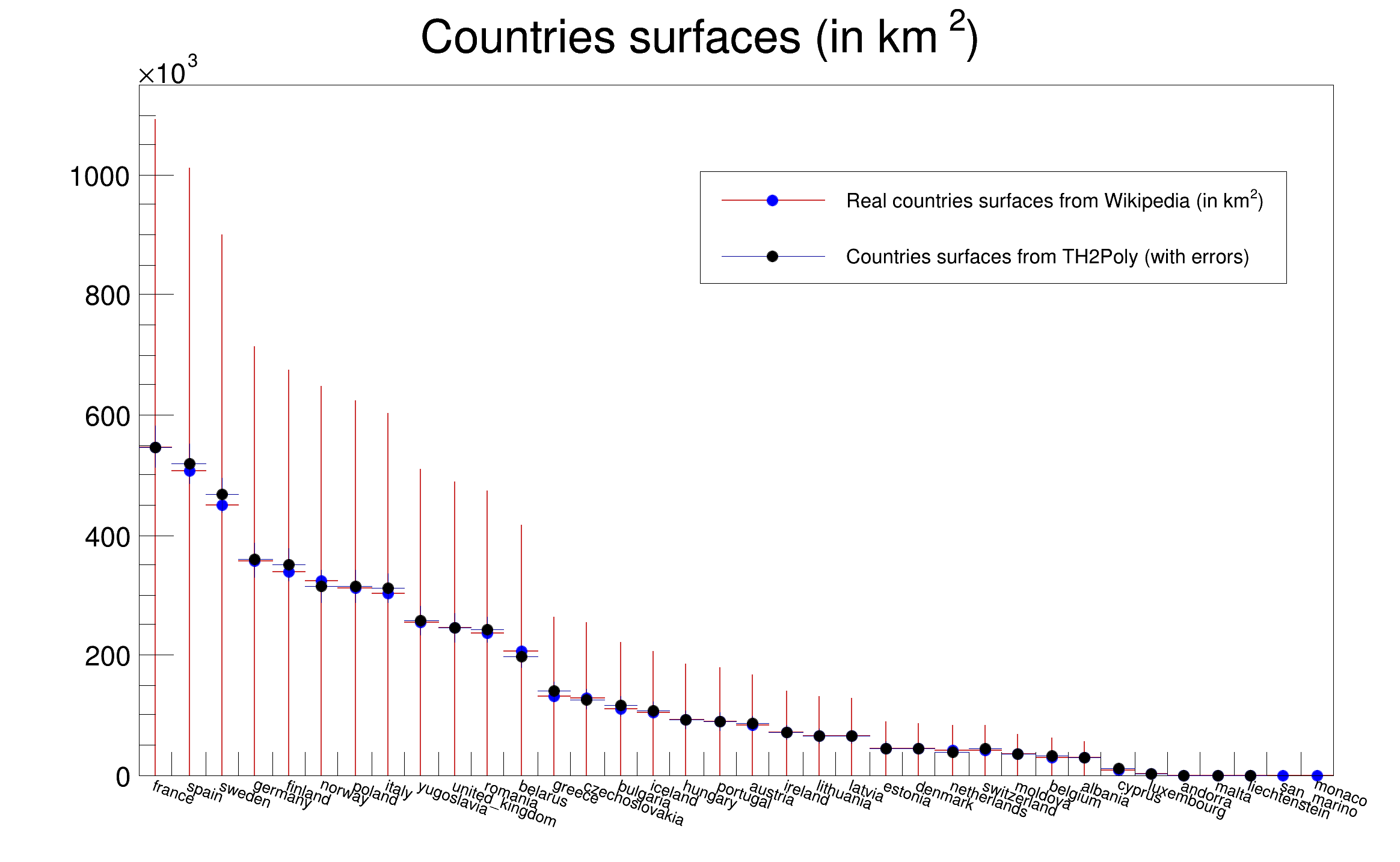ROOT   Reference Guideth2polyEurope.C File Reference

## Detailed DescriptionThis tutorial illustrates how to create an histogram with polygonal bins (TH2Poly), fill it and draw it.

The initial data are stored in TMultiGraphs. They represent the european countries. The histogram filling is done according to a Mercator projection, therefore the bin contains should be proportional to the real surface of the countries.

The initial data have been downloaded from: http://www.maproom.psu.edu/dcw/ This database was developed in 1991/1992 and national boundaries reflect political reality as of that time.

The script is shooting npoints (script argument) randomly over the Europe area. The number of points inside the countries should be proportional to the country surface The estimated surface is compared to the surfaces taken from wikipedia.Partitioning: Real Time = 1.30 seconds Cpu Time = 1.31 seconds
Filling : Real Time = 4.01 seconds Cpu Time = 4.00 seconds
THPoly Europe surface estimation error wrt wikipedia = 1.260096 per cent when using 500000 points
void th2polyEurope(Int_t npoints=500000)
{
Int_t i,j;
Double_t lon1 = -25;
Double_t lon2 = 35;
Double_t lat1 = 34;
Double_t lat2 = 72;
Double_t R = (lat2-lat1)/(lon2-lon1);
Int_t W = 800;
Int_t H = (Int_t)(R*800);
gStyle->SetStatX(0.28);
gStyle->SetStatY(0.45);
gStyle->SetStatW(0.15);
// Canvas used to draw TH2Poly (the map)
TCanvas *ce = new TCanvas("ce", "ce",0,0,W,H);
ce->SetGridx();
ce->SetGridy();
// Real surfaces taken from Wikipedia.
const Int_t nx = 36;
// see http://en.wikipedia.org/wiki/Area_and_population_of_European_countries
const char *countries[nx] = {
"france", "spain", "sweden", "germany", "finland",
"norway", "poland", "italy", "yugoslavia", "united_kingdom",
"romania", "belarus","greece", "czechoslovakia","bulgaria",
"iceland", "hungary","portugal","austria", "ireland",
"lithuania", "latvia", "estonia", "denmark", "netherlands",
"switzerland","moldova","belgium", "albania", "cyprus",
"luxembourg", "andorra","malta", "liechtenstein", "san_marino", "monaco" };
Float_t surfaces[nx] = {
547030, 505580, 449964, 357021, 338145,
324220, 312685, 301230, 255438, 244820,
237500, 207600, 131940, 127711, 110910,
103000, 93030, 89242, 83870, 70280,
65200, 64589, 45226, 43094, 41526,
41290, 33843, 30528, 28748, 9250,
2586, 468, 316, 160, 61, 2};
TH1F *h = new TH1F("h","Countries surfaces (in km^{2})",3,0,3);
for (i=0; i<nx; i++) h->Fill(countries[i], surfaces[i]);
h->LabelsDeflate();
TFile *f;
if (!f) {
printf("Cannot access europe.root. Is internet working ?\n");
return;
}
TH2Poly *p = new TH2Poly(
"Europe",
"Europe (bin contents are normalized to the surfaces in km^{2})",
lon1,lon2,lat1,lat2);
p->GetXaxis()->SetNdivisions(520);
p->GetXaxis()->SetTitle("longitude");
p->GetYaxis()->SetTitle("latitude");
p->SetContour(100);
TKey *key;
TIter nextkey(gDirectory->GetListOfKeys());
while ((key = (TKey*)nextkey())) {
if (obj->InheritsFrom("TMultiGraph")) {
mg = (TMultiGraph*)obj;
}
}
Double_t longitude, latitude;
Double_t x, y, pi4 = TMath::Pi()/4, alpha = TMath::Pi()/360;
gBenchmark->Start("Partitioning");
p->ChangePartition(100, 100);
gBenchmark->Show("Partitioning");
// Fill TH2Poly according to a Mercator projection.
gBenchmark->Start("Filling");
for (i=0; i<npoints; i++) {
longitude = r.Uniform(lon1,lon2);
latitude = r.Uniform(lat1,lat2);
x = longitude;
y = 38*TMath::Log(TMath::Tan(pi4+alpha*latitude));
p->Fill(x,y);
}
gBenchmark->Show("Filling");
Int_t nbins = p->GetNumberOfBins();
Double_t maximum = p->GetMaximum();
// h2 contains the surfaces computed from TH2Poly.
TH1F *h2 = (TH1F *)h->Clone("h2");
h2->Reset();
for (j=0; j<nx; j++) {
for (i=0; i<nbins; i++) {
if (strstr(countries[j],p->GetBinName(i+1))) {
h2->Fill(countries[j],p->GetBinContent(i+1));
h2->SetBinError(j, p->GetBinError(i+1));
}
}
}
// Normalize the TH2Poly bin contents to the real surfaces.
Double_t scale = surfaces/maximum;
for (i=0; i<nbins; i++) p->SetBinContent(i+1, scale*p->GetBinContent(i+1));
p->Draw("COLZ");
TCanvas *c1 = new TCanvas("c1", "c1",W+10,0,W-20,H);
c1->SetRightMargin(0.047);
scale = h->GetMaximum()/h2->GetMaximum();
h->SetStats(0);
h->SetLineColor(kRed-3);
h->SetLineWidth(2);
h->SetMarkerStyle(20);
h->SetMarkerColor(kBlue);
h->SetMarkerSize(0.8);
h->Draw("LP");
h->GetXaxis()->SetLabelFont(42);
h->GetXaxis()->SetLabelSize(0.03);
h->GetYaxis()->SetLabelFont(42);
h2->Scale(scale);
Double_t scale2=TMath::Sqrt(scale);
for (i=0; i<nx; i++) h2->SetBinError(i+1, scale2*h2->GetBinError(i+1));
h2->Draw("E SAME");
h2->SetMarkerStyle(20);
h2->SetMarkerSize(0.8);
TLegend *leg = new TLegend(0.5,0.67,0.92,0.8,NULL,"NDC");
leg->SetTextFont(42);
leg->SetTextSize(0.025);
leg->AddEntry(h,"Real countries surfaces from Wikipedia (in km^{2})","lp");
leg->AddEntry(h2,"Countries surfaces from TH2Poly (with errors)","lp");
leg->Draw();
leg->Draw();
Double_t wikiSum = h->Integral();
Double_t polySum = h2->Integral();
Double_t error = TMath::Abs(wikiSum-polySum)/wikiSum;
printf("THPoly Europe surface estimation error wrt wikipedia = %f per cent when using %d points\n",100*error,npoints);
}
ROOT::R::TRInterface & r
Definition: Object.C:4
#define f(i)
Definition: RSha256.hxx:104
#define h(i)
Definition: RSha256.hxx:106
int Int_t
Definition: RtypesCore.h:45
double Double_t
Definition: RtypesCore.h:59
float Float_t
Definition: RtypesCore.h:57
@ kRed
Definition: Rtypes.h:66
@ kBlue
Definition: Rtypes.h:66
R__EXTERN TBenchmark * gBenchmark
Definition: TBenchmark.h:59
#define gDirectory
Definition: TDirectory.h:290
R__EXTERN TStyle * gStyle
Definition: TStyle.h:412
virtual void SetNdivisions(Int_t n=510, Bool_t optim=kTRUE)
Set the number of divisions for this axis.
Definition: TAttAxis.cxx:228
virtual void SetMarkerStyle(Style_t mstyle=1)
Set the marker style.
Definition: TAttMarker.h:40
virtual void SetMarkerSize(Size_t msize=1)
Set the marker size.
Definition: TAttMarker.h:41
virtual void Start(const char *name)
Starts Benchmark with the specified name.
Definition: TBenchmark.cxx:172
virtual void Show(const char *name)
Stops Benchmark name and Prints results.
Definition: TBenchmark.cxx:155
The Canvas class.
Definition: TCanvas.h:23
virtual void ToggleEventStatus()
Toggle event statusbar.
Definition: TCanvas.cxx:2446
A ROOT file is a suite of consecutive data records (TKey instances) with a well defined format.
Definition: TFile.h:54
static Bool_t SetCacheFileDir(ROOT::Internal::TStringView cacheDir, Bool_t operateDisconnected=kTRUE, Bool_t forceCacheread=kFALSE)
Definition: TFile.h:324
static TFile * Open(const char *name, Option_t *option="", const char *ftitle="", Int_t compress=ROOT::RCompressionSetting::EDefaults::kUseCompiledDefault, Int_t netopt=0)
Create / open a file.
Definition: TFile.cxx:3997
1-D histogram with a float per channel (see TH1 documentation)}
Definition: TH1.h:575
virtual void Reset(Option_t *option="")
Reset.
Definition: TH1.cxx:9947
virtual Double_t GetBinError(Int_t bin) const
Return value of error associated to bin number bin.
Definition: TH1.cxx:8903
TAxis * GetXaxis()
Definition: TH1.h:320
virtual Double_t GetMaximum(Double_t maxval=FLT_MAX) const
Return maximum value smaller than maxval of bins in the range, unless the value has been overridden b...
Definition: TH1.cxx:8390
virtual void SetBinError(Int_t bin, Double_t error)
Set the bin Error Note that this resets the bin eror option to be of Normal Type and for the non-empt...
Definition: TH1.cxx:9046
virtual Int_t Fill(Double_t x)
Increment bin with abscissa X by 1.
Definition: TH1.cxx:3350
TAxis * GetYaxis()
Definition: TH1.h:321
virtual void SetContour(Int_t nlevels, const Double_t *levels=0)
Set the number and values of contour levels.
Definition: TH1.cxx:8331
virtual Double_t Integral(Option_t *option="") const
Return integral of bin contents.
Definition: TH1.cxx:7834
virtual void Draw(Option_t *option="")
Draw this histogram with options.
Definition: TH1.cxx:3073
virtual void Scale(Double_t c1=1, Option_t *option="")
Multiply this histogram by a constant c1.
Definition: TH1.cxx:6564
2D Histogram with Polygonal Bins
Definition: TH2Poly.h:66
Int_t GetNumberOfBins() const
Definition: TH2Poly.h:110
virtual Int_t Fill(Double_t x, Double_t y)
Increment the bin containing (x,y) by 1.
Definition: TH2Poly.cxx:589
void ChangePartition(Int_t n, Int_t m)
Changes the number of partition cells in the histogram.
Definition: TH2Poly.cxx:440
const char * GetBinName(Int_t bin) const
Returns the bin name.
Definition: TH2Poly.cxx:822
virtual Double_t GetBinContent(Int_t bin) const
Returns the content of the input bin For the overflow/underflow/sea bins:
Definition: TH2Poly.cxx:765
Adds a new bin to the histogram.
Definition: TH2Poly.cxx:222
virtual Double_t GetBinError(Int_t bin) const
Returns the value of error associated to bin number bin.
Definition: TH2Poly.cxx:780
virtual void SetBinContent(Int_t bin, Double_t content)
Sets the contents of the input bin to the input content Negative values between -1 and -9 are for the...
Definition: TH2Poly.cxx:1280
Double_t GetMaximum() const
Returns the maximum value of the histogram.
Definition: TH2Poly.cxx:842
Book space in a file, create I/O buffers, to fill them, (un)compress them.
Definition: TKey.h:28
To read a TObject* from the file.
Definition: TKey.cxx:750
This class displays a legend box (TPaveText) containing several legend entries.
Definition: TLegend.h:23
A TMultiGraph is a collection of TGraph (or derived) objects.
Definition: TMultiGraph.h:36
virtual void SetTitle(const char *title="")
Set the title of the TNamed.
Definition: TNamed.cxx:164
Mother of all ROOT objects.
Definition: TObject.h:37
virtual Bool_t InheritsFrom(const char *classname) const
Returns kTRUE if object inherits from class "classname".
Definition: TObject.cxx:445
void SetGridx(Int_t value=1) override
void SetGridy(Int_t value=1) override
This is the base class for the ROOT Random number generators.
Definition: TRandom.h:27
void SetOptStat(Int_t stat=1)
The type of information printed in the histogram statistics box can be selected via the parameter mod...
Definition: TStyle.cxx:1589
void SetStatX(Float_t x=0)
Definition: TStyle.h:380
void SetStatW(Float_t w=0.19)
Definition: TStyle.h:382
void SetStatY(Float_t y=0)
Definition: TStyle.h:381
return c1
Definition: legend1.C:41
Double_t y[n]
Definition: legend1.C:17
Double_t x[n]
Definition: legend1.C:17
leg
Definition: legend1.C:34
#define H(x, y, z)
static constexpr double mg
Double_t Log(Double_t x)
Definition: TMath.h:760
Double_t Sqrt(Double_t x)
Definition: TMath.h:691
constexpr Double_t Pi()
Definition: TMath.h:37
Double_t Tan(Double_t)
Definition: TMath.h:647
Short_t Abs(Short_t d)
Definition: TMathBase.h:120

Definition in file th2polyEurope.C.